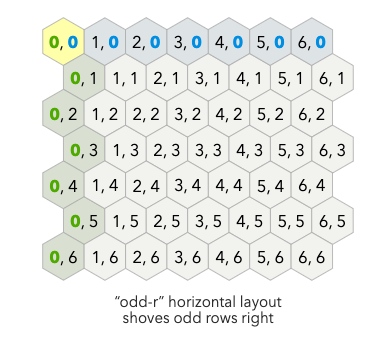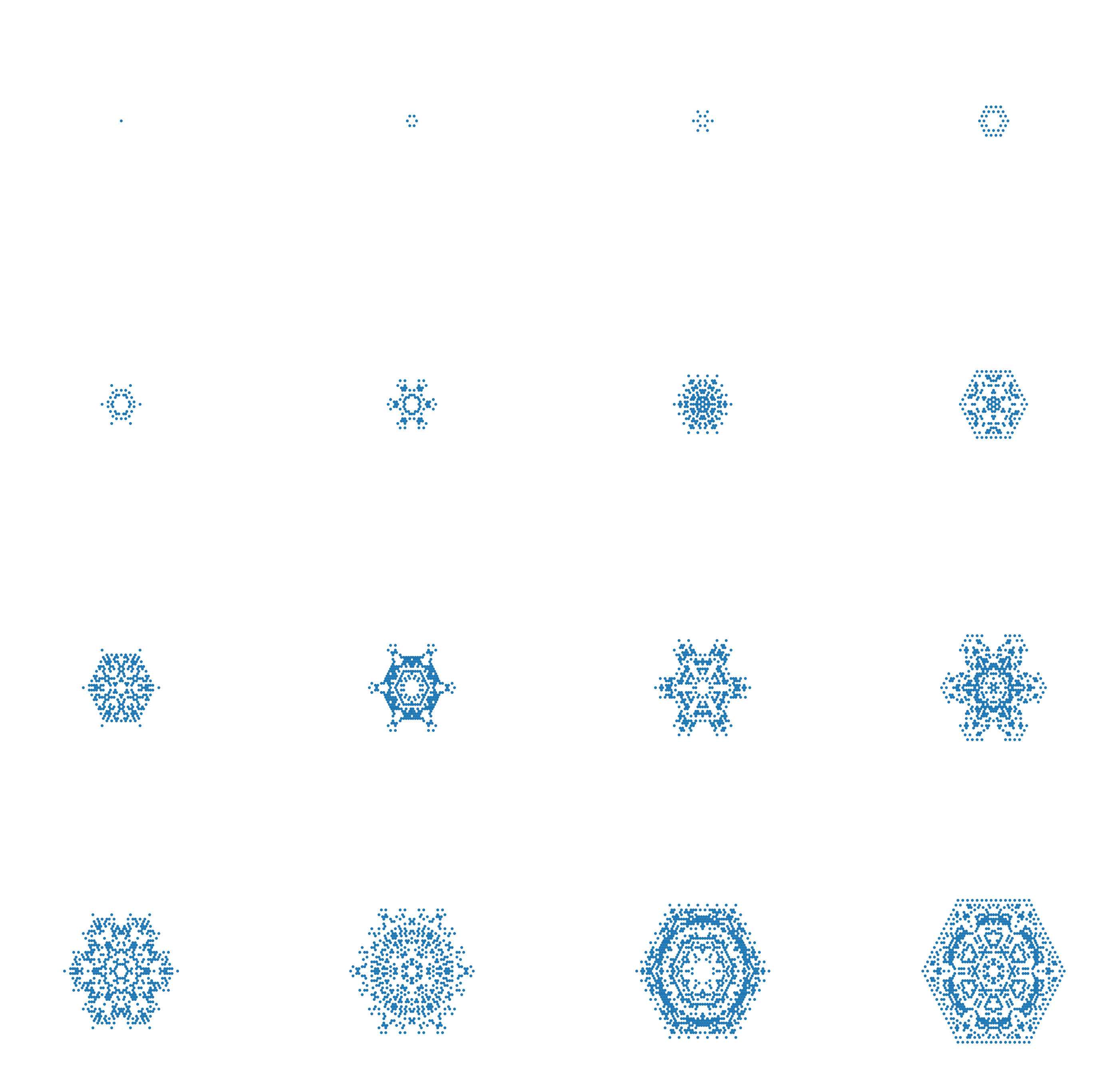# Making snowflakes with cellular automata ❄️

TL;DR: Generate snowflakes using cellular automata.

Click on the image to reset the animation!

## Making Snowflakes

Arguably, the most famous Cellular Automata (CA) is Conway’s Game of Life. What’s interesting is that we can slightly modify the rules to generate snowlakes!

In the original CA, we work on a square grid and assume each cell to have 8 neighbors. For our snowflake, we will work on a hexagonal lattice and assume each cell to have 6 neighbors.

We will be using the `odd-r` representation to represent our lattice (see this wonderful post for more info). This allows us to use a regular array and simply “convert” the representation to a hexagonal grid.To get a cell’s neighboring cell coordinates, we can then simply implement the following function:

``````def get_adjacent_cells(i, j):
"""Given a position i,j, get all adjacent neighbors on hexagonal grid."""
if j % 2 == 0:
adjacent_cells =  (i-1, j-1), (i, j-1), (i-1, j), (i+1, j), (i-1, j+1), (i, j+1)
else:
adjacent_cells =  (i, j-1), (i+1, j-1), (i-1, j), (i+1, j), (i, j+1), (i+1, j+1)
``````

We then need to define an update rule for our snowflake CA. We keep track of two states:

• `"ice": 1`
• `"melted": 0`

To define an update, we simply count the number of “icy” and “melted” neighbors for a given cell and decide what should then happen:

Here’s an example set of rules:

``````if sum(neighbors) == 1:
snowflake[i, j] = 1
elif sum(neighbors) == 2:
snowflake[i, j] = 0
elif sum(neighbors) == 3:
snowflake[i, j] = 0
elif sum(neighbors) == 4:
snowflake[i, j] = 1
elif sum(neighbors) == 5:
snowflake[i, j] = 1
elif sum(neighbors) == 6:
snowflake[i, j] = 0
``````

Now we can simply iterate over this and generate pretty snowflakes!Tags:

Categories:

Updated: